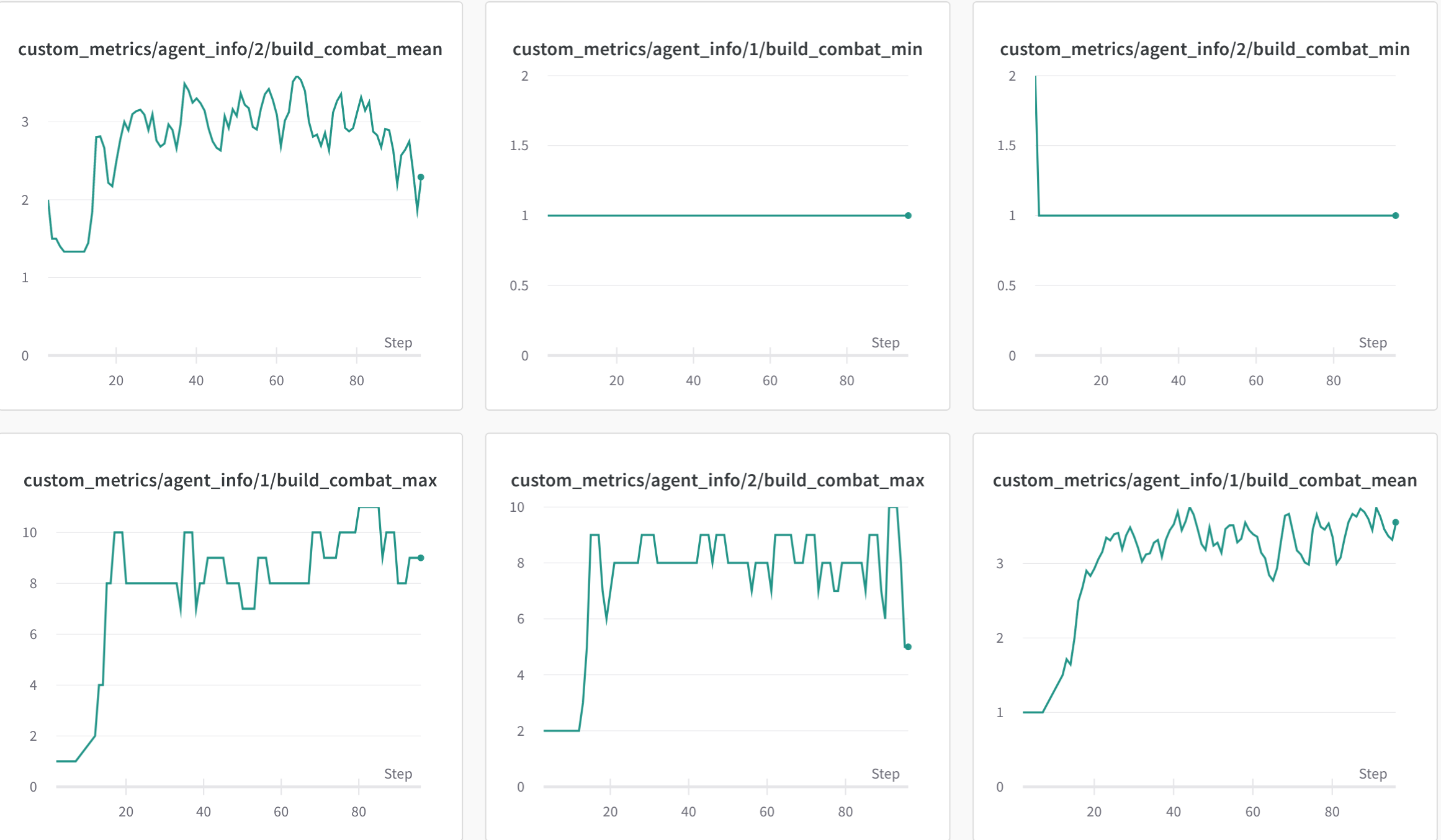# Reinforcement Learning with RLLib

Griddly provides support for reinforcement learning using the RLLib reinforcement learning library.

While RLLib doesn’t support OpenAI Gym registered environments, it does provide a similar interface which is supported by Griddly’s `RLLibEnv` environment.

Griddly provides two classes, `RLLibEnv` and `RLLibMultiAgentWrapper` which abstract away all the tedious parts of wrapping environments for RL and leaves you to concentrate on training algorithms, designing networks and game mechanics.

Examples for single-agent and multi-agent training are provided.

Warning

All examples and networks are implemented using PyTorch. Some examples may be modified to work with Tensorflow, but we do not provide explicit support for Tensorflow.

## Examples Setup

Griddly installs most of the dependencies for you automatically when it is installed, however you will need to install RLLlib and Pytorch to run the provided examples.

You can install RLLib and pytorch using the following command:

```pip install ray[rllib]==1.2.0 torch==1.8.0
```

All RLLib examples can be found in `python/examples/rllib/`

## Environment Parameters

Parameters for the environments, such as the GDY file for the game and Observer options can be sent to the environment using the `env_config` dictionary.

Most of the parameters here are the same as the parameters that can be given to the `gym.make()` command when creating a Griddly environment for OpenAI Gym.

```'env_config': {
'yaml_file': 'Single-Player/GVGAI/clusters_partially_observable.yaml',

'global_observer_type': gd.ObserverType.SPRITE_2D,
'record_video_config': {
'frequency': 100000
},

'random_level_on_reset': True,
'max_steps': 1000,
},
```

The above example will also record a video of the environment (rendered using the `SPRITE_2D` renderer) for one episode every 100000 steps. Finally the max_steps of the environment will be override to be 1000 steps before the environment is reset automatically.

## Level Randomization

Partially observable games have a fixed observations space regardless of the size of the levels. Additionally several games have levels of fixed size.

With these games, the level can be randomized at the end of every episode using the `random_level_on_reset` option in the `env_config` section of RLLib’s config.

```'env_config': {

'random_level_on_reset': True,

...
```

If this is set to true then the agent will be placed in one of the random levels described in the GDY file each time the episode restarts.

## Agents

We provide a few custom agent models that can be used with any Griddly environment.

### Simple Convolutional agent

The simple convolutional agent stacks three convolutional layers that preserve the size of the input. After these layers the representation is flattened and linear layers are then used for the actor and critic heads.

To use the simple `SimpleConvAgent, register the custom model with RLLib and then use it in your training ``config`:

```ModelCatalog.register_custom_model('SimpleConv', SimpleConvAgent)

...

config = {

'model': {
'custom_model': 'SimpleConv'
'custom_model_config': .....
}

...

}
```

#### SimpleConvAgent

```class SimpleConvAgent(TorchModelV2, nn.Module):
"""
Simple Convolution agent that calculates the required linear output layer
"""

def __init__(self, obs_space, action_space, num_outputs, model_config, name):
super().__init__(obs_space, action_space, num_outputs, model_config, name)
nn.Module.__init__(self)

self._num_objects = obs_space.shape
self._num_actions = num_outputs

linear_flatten = np.prod(obs_space.shape[:2])*64

self.network = nn.Sequential(
nn.ReLU(),
nn.ReLU(),
nn.Flatten(),
layer_init(nn.Linear(linear_flatten, 1024)),
nn.ReLU(),
layer_init(nn.Linear(1024, 512)),
nn.ReLU(),
)

layer_init(nn.Linear(512, 256), std=0.01),
nn.ReLU(),
layer_init(nn.Linear(256, self._num_actions), std=0.01)
)

layer_init(nn.Linear(512, 1), std=0.01)
)

def forward(self, input_dict, state, seq_lens):
obs_transformed = input_dict['obs'].permute(0, 3, 1, 2)
network_output = self.network(obs_transformed)
self._value = value.reshape(-1)
return logits, state

def value_function(self):
return self._value
```

### Global Average Pooling

Griddly environments’ observation spaces differ between games, levels and visualization options. In order to handle this in a generic way using neural networks, we provide a Global Average Pooling agent GAPAgent, which can be used with any 2D environment with no additional configuration.

All you need to do is register the custom model with RLLib and then use it in your training `config`:

```ModelCatalog.register_custom_model('GAP', GAPAgent)

...

config = {

'model': {
'custom_model': 'GAP'
'custom_model_config': .....
}

...

}
```

#### GAPAgent

```class GAPAgent(TorchModelV2, nn.Module):
"""
Global Average Pooling Agent
This is the same agent used in https://arxiv.org/abs/2011.06363.

Global average pooling is a simple way to allow training grid-world environments regardless o the size of the grid.
"""

def __init__(self, obs_space, action_space, num_outputs, model_config, name):
super().__init__(obs_space, action_space, num_outputs, model_config, name)
nn.Module.__init__(self)

self._num_objects = obs_space.shape

self._num_actions = num_outputs

self.network = nn.Sequential(
nn.ReLU(),
nn.ReLU(),
GlobalAvePool(2048),
layer_init(nn.Linear(2048, 1024)),
nn.ReLU(),
layer_init(nn.Linear(1024, 512)),
nn.ReLU(),
)

layer_init(nn.Linear(512, 256), std=0.01),
nn.ReLU(),
layer_init(nn.Linear(256, self._num_actions), std=0.01)
)

layer_init(nn.Linear(512, 1), std=0.01)
)

def forward(self, input_dict, state, seq_lens):
obs_transformed = input_dict['obs'].permute(0, 3, 1, 2)
network_output = self.network(obs_transformed)
self._value = value.reshape(-1)
return logits, state

def value_function(self):
return self._value
```

You can read more about agents that use Global Average Pooling here: https://arxiv.org/abs/2005.11247

## Recording Videos

Griddly can automatically record videos during training by placing the `record_video_config` dictionary into the standard RLLib `env_config`.

```'env_config':
'record_video_config': {
'frequency': 20000
'directory': '/home/griddlyuser/my_experiment_videos'
'include_global': True,
'include_agents': False,
},

...
}
```

Warning

the `directory` value must be an absolute path, as the working directory of workers is controlled by Ray.

Videos can be recorded from the perspective of the agent and the perspective of the global observer. `include_global` and `include_agents` will set which videos are recorded.

The triggering of videos is configured using the `frequency` variable. The `frequency` variable refers to the number of steps in each environment that pass before the recording is triggered.

Once triggered, the next episode is recorded in full. Videos of episodes are recorded on the first environment in every worker in RLLib.

To automatically upload videos to WandB, the `VideoCallback` can be set in the RLLib config:

```'config': {
...,

'callbacks': VideoCallback,

...
}
```

## Recording Environment ActionsAn example of logged events for each agent in an environment during training. Can help to diagnose problems with reward shaping and track exploration.

Griddly’s RLLib integration hooks into the Event History and records all the frequency of the actions that are being taken by agents during training. This event history can then be picked up in the agent’s `info` in RLLib’s callback methods, e,g `on_episode_step`

```'env_config':
'record_actions': True,

...
}
```

To automatically upload action events to WandB, the `ActionTrackerCallback` can be set in the RLLib config:
```'config': {